# Number Puzzles - Questions with Answers for SSC Exam | Page-2

Questions

### Find the missing term in each of the following:

8A 13
B 15
C 16
D 19

Solution:

The sum of the two numbers in the upper part is seven times the number in the lower part.

i.e. (25 + 17) ÷ 7 = 6

(38 + 18) ÷ 7 = 8

So, the missing number = (89 + 16) ÷ 7 = 105 ÷ 7 = 15

9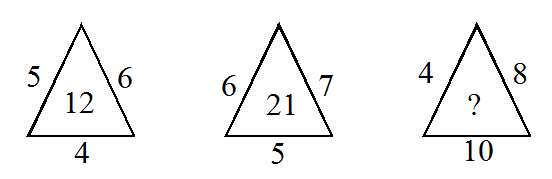A 30
B 32
C 34
D 36

Solution:

(5 × 6 × 4) ÷ 10 = 12

(6 × 7 × 5) ÷ 10 = 21

So, missing number = (4 × 8 × 10) ÷ 10 = 32

10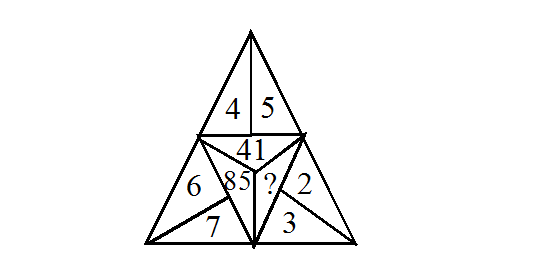A 13
B 15
C 5
D 10

Solution:

52 + 42 = 41

62 + 72 = 85

Hence, missing number = 22 + 32 = 4 + 9 = 13

11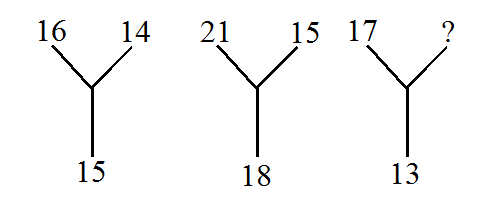A 13
B 7
C 11
D 9

Solution:

(16 + 14) ÷ 2 = 30 ÷ 2 = 15

(21 + 15) ÷ 2 = 36 ÷ 2 = 18

So, (17 + x) ÷ 2 = 13 ⇒ 17 + x = 26 ⇒ x = 26 - 17 ⇒ x = 9

12A 1
B 2
C 3
D 4

Solution:

The number at the bottom is HCF of the rest of the number.

So, missing number is = HCF of 2, 7 and 8 = 1

13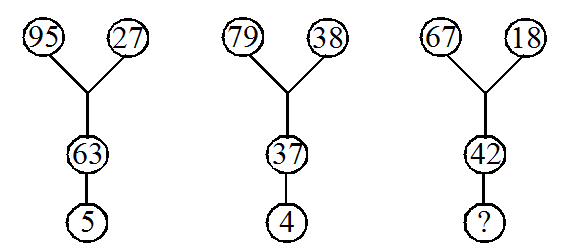A 5
B 7
C 12
D 15

Solution:

95 - (27 + 63) = 5

79 - (38 + 37) = 4

So, missing number = 67 - (18 + 42) = 7

14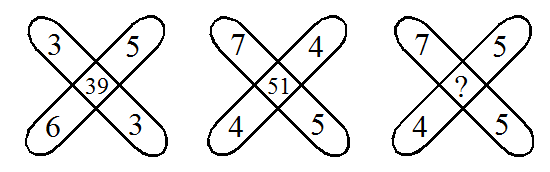A 41
B 45
C 53
D 55

Solution:

39 = (3 × 3) + (6 × 5)

51 = (4 × 4) + (5 × 7)

So, missing number = (7 × 5) + (4 × 5) = 55